A Follow-on to Previous Posts

This is a follow-on post to my post on Assistive Technologies: Math Software for Supporting People with Dysgraphia. In this post I will provide a step-by-step explanation on how to use equations from Efofex Software's FX Equation software with LibreOffice's Writer program.

How to Insert an Equation from FX Equation into LibreOffice Writer

There are two methods for inserting an equation from FX Equation into LibreOffice Writer:

1. Insert the equation as a simple picture (easy, but not flexible) or
2. Insert the equation as a LibreOffice Formula (a little harder, but can be edited later).

Inserting an Equation from FX Equation as a Picture/Figure

The first approach is fairly simple. After you have your equation ready to copy, select the `Copy` button or type `Ctrl-C` and then open up LibreOffice Writer and type `Ctrl-V` (or Select `Paste` from the `Edit` menu, etc.) to paste the equation into the document like any picture.

The following picture shows the `Copy` button highlighted in light blue between the `Cut` button (the scissors) and the `Copy As` button.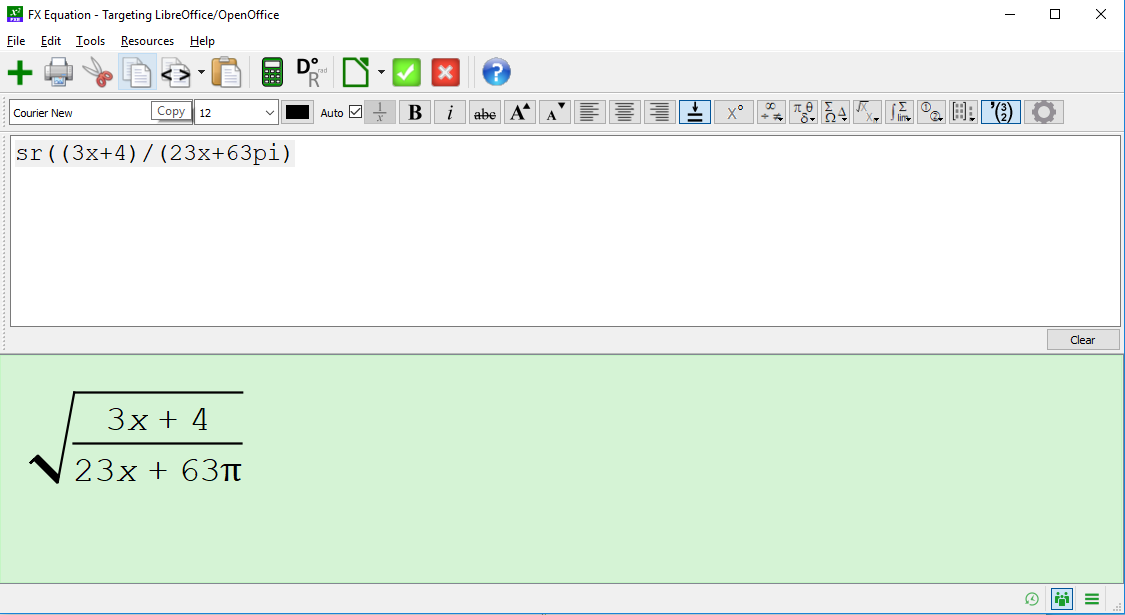I find it easiest if the picture is "anchored" to the page as a character. That way it flows around in the document like regular text and does not just float mysteriously on the page. To do this, you:

1. Right-click on the picture.
3. Select "As Character".

The following picture illustrates this menu from LibreOffice Writer: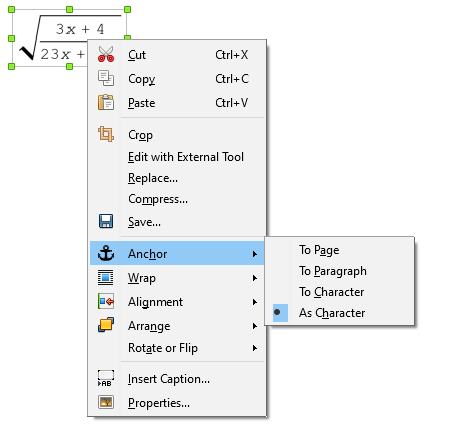Inserting an Equation from FX Equation as a LibreOffice Formula

So there are probably several methods for doing this. I'll brake it up into a few options.

Option 1: Using the Green Check Mark in FX Equation

One approach is to set the target program that is going to "receive" the equation. In our case LibreOffice is the target, so you can select `Target LibreOffice/OpenOffice` as the target as shown in this picture: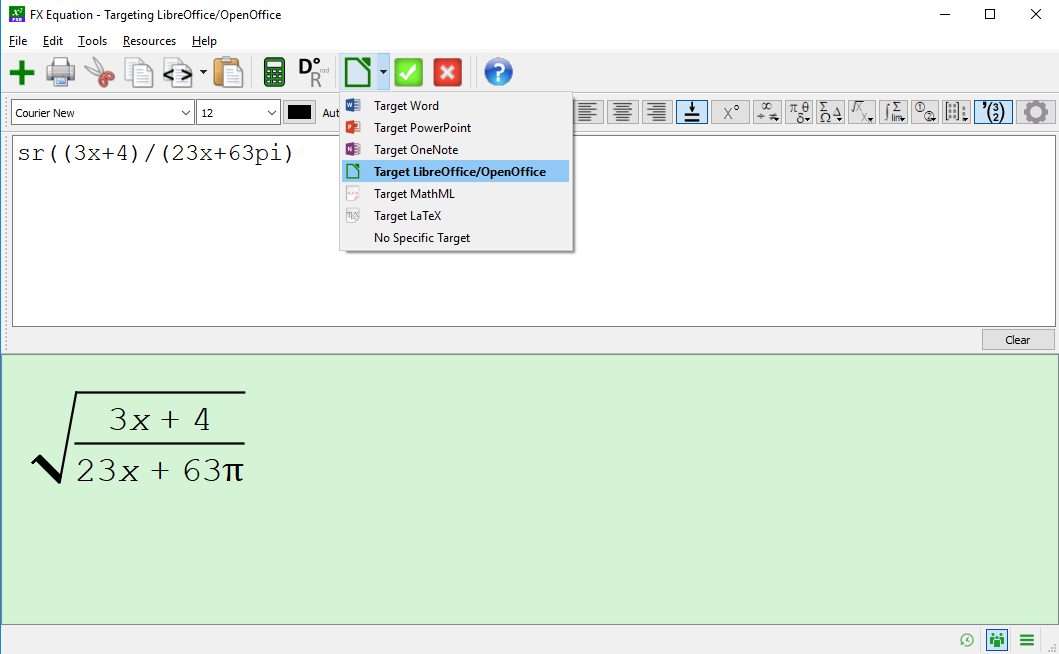Once you do this, you can just select the large green check mark on the button bar and the equation will be provided in LibreOffice Equation/Formula format onto the Windows clipboard and the FX Equation editor will hide itself while you work with LibreOffice (though, it is still running).

Option 2: Using Copy As LibreOffice/OpenOffice Equation

In this method, you can click on the little downward pointing triangle on the `Copy As` button on the button bar (the one in between the `Paste` button that has a clipboard on it and the simple `Copy` button). A little menu will pop up where you can select ```Copy As LibreOffice/OpenOffice Equation```. The following figure illustrates this approach: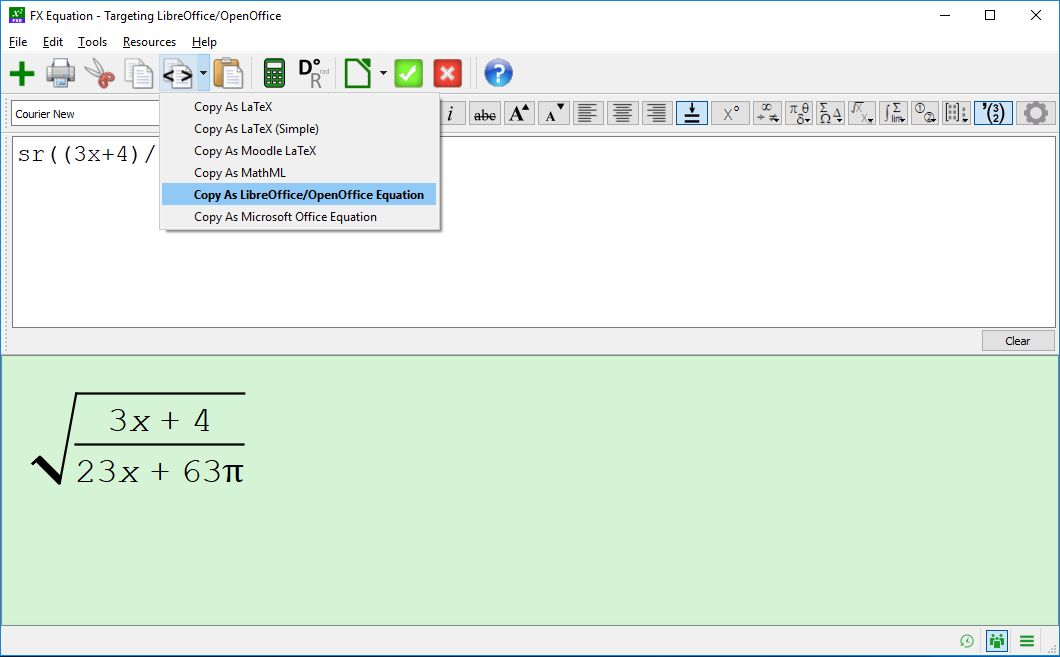At this point, the equation is on the clipboard ready to go.

Once you have selected the `Copy As LibreOffice/OpenOffice Equation` once, the next time you click the larger `Copy As` button itself, it will remember your last setting (in this case ```Copy As LibreOffice/OpenOffice Equation```).

Finishing the Job: Pasting the Equation into LibreOffice Writer

Once the equation is on the clipboard using one of the two methods just discussed, the next thing to do is to go to the LibreOffice Writer document you want to insert the equation into and do the following:

1. Click the location in the LibreOffice Writer document where you want to insert the equation/formula.
2. From the `Insert` menu, select `Object` and then `Formula...`. See the following picture for an illustration:3. At that point the Formula/Equation editor pops up. In the bottom right box (highlighted in green in the picture below), you can now paste the equation.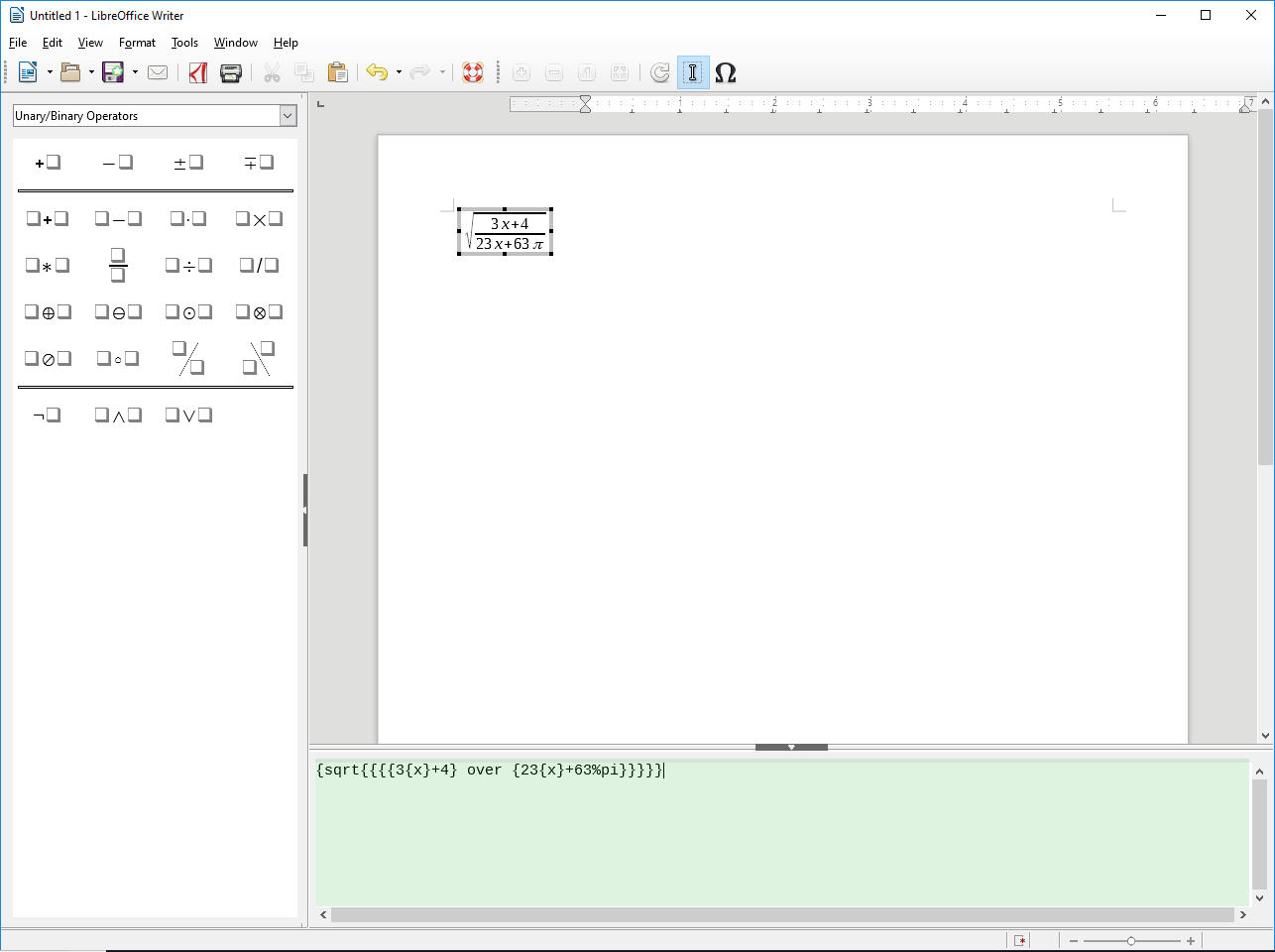4. To get out of the Formula/Equation editor, click just about anywhere in the document other than the equation itself.

To edit the fonts or contents of the Formula, you can double click on the Formula to open the Formula/Equation editor again.